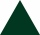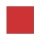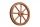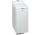# Cone

Circular cone of height 15 cm and volume 5699 cm3 is at one-third of the height (measured from the bottom) cut by a plane parallel to the base. Calculate the radius and circumference of the circular cut.

Result

r =  12.7 cm
o =  79.8 cm

#### Solution:

$V = 5699 \ cm^3 \ \\ h = 15 \ cm \ \\ \ \\ V = \dfrac{ 1 }{ 3 } \cdot \ \pi \cdot \ r_{ 0 }^2 \cdot \ h \ \\ \ \\ r_{ 0 } = \sqrt{ \dfrac{ 3 \cdot \ V }{ \pi \cdot \ h } } = \sqrt{ \dfrac{ 3 \cdot \ 5699 }{ 3.1416 \cdot \ 15 } } \doteq 19.0476 \ cm \ \\ \ \\ r = \dfrac{ 2 }{ 3 } \cdot \ r_{ 0 } = \dfrac{ 2 }{ 3 } \cdot \ 19.0476 \doteq 12.6984 = 12.7 \ \text { cm }$
$o = 2 \pi \cdot \ r = 2 \cdot \ 3.1416 \cdot \ 12.6984 \doteq 79.7965 = 79.8 \ \text { cm }$

Leave us a comment of this math problem and its solution (i.e. if it is still somewhat unclear...):Math student
The solution that you give us doesn't make sense.Dr Math#### Following knowledge from mathematics are needed to solve this word math problem:

Need help calculate sum, simplify or multiply fractions? Try our fraction calculator. Do you want to convert length units? Tip: Our volume units converter will help you with the conversion of volume units. See also our trigonometric triangle calculator.

## Next similar math problems:

1. Circle arcCircle segment has a circumference of 135.26 dm and 2096.58 dm2 area. Calculate the radius of the circle and size of central angle.
2. CylindersArea of the side of two cylinders is same rectangle of 33 mm × 18 mm. Which cylinder has a larger volume and by how much?
3. StepsHow many steps you save if you go square estate for diagonal (crosswise), rather than circumvent on the two sides of its perimeter with 307 steps.
4. An equilateral triangleThe perimeter of an equilateral triangle is 33cm. How long is each side?Calculate the radius of the circle whose length is 107 cm larger than its diameter
6. Square diagonalCalculate length of the square diagonal if the perimeter is 476 cm.
7. CalculateCalculate the square area if its perimeter is 14dm.
8. Coal mineThe towing wheel has a diameter of 1.7 meters. How many meters does the elevator cage lower when the wheel turns 32 times?
9. Trapezoid MOThe rectangular trapezoid ABCD with right angle at point B, |AC| = 12, |CD| = 8, diagonals are perpendicular to each other. Calculate the perimeter and area of ​​the trapezoid.
10. GardenArea of a square garden is 6/4 of triangle garden with sides 56 m, 35 m, and 35 m. How many meters of fencing need to fence a square garden?
11. Triangle SASCalculate the area and perimeter of the triangle, if the two sides are 51 cm and 110 cm long and angle them clamped is 130 °.
12. RhombusCalculate the perimeter and area of ​​a rhombus whose diagonals are 39 cm and 51 cm long.
13. WasherWashing machine drum wash at 58 RPM. Washing machine motor pulley has diameter 9 cm. What must be the diameter of the drum machine pulley when the motor is at 438 RPM?
14. Right triangle AlefThe obvod of a right triangle is 84 cm, the hypotenuse is 37 cm long. Determine the lengths of the legs.
15. RectangleIn rectangle with sides, 6 and 3 mark the diagonal. What is the probability that a randomly selected point within the rectangle is closer to the diagonal than to any side of the rectangle?
16. Two squaresTwo squares whose sides are in the ratio 5:2 have sum of its perimeters 73 cm. Calculate the sum of area this two squares.
17. Rectangle - sides ratioCalculate area of rectangle whose sides are in ratio 3:13 and perimeter is 673.